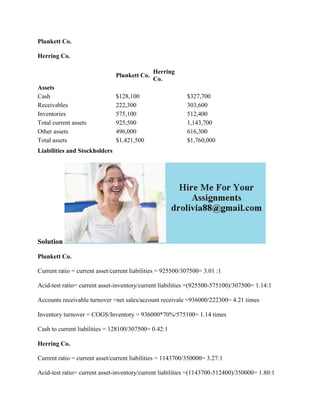Successfully reported this slideshow.

# Plunkett Co- Herring Co- Plunkett Co- Herring Co- Assets.docx×

# Plunkett Co- Herring Co- Plunkett Co- Herring Co- Assets.docx

Plunkett Co.
Herring Co.
Solution
Plunkett Co.
Current ratio = current asset/current liabilities = 925500/307500= 3.01 :1
Acid-test ratio= current asset-inventory/current liabilities =(925500-575100)/307500= 1.14:1
Accounts receivable turnover =net sales/account receivale =936000/222300= 4.21 times
Inventory turnover = COGS/Inventory = 936000*70%/575100= 1.14 times
Cash to current liabilities = 128100/307500= 0.42:1
Herring Co.
Current ratio = current asset/current liabilities = 1143700/350000= 3.27:1
Acid-test ratio= current asset-inventory/current liabilities =(1143700-512400)/350000= 1.80:1
Accounts receivable turnover =net sales/account receivale =1516200/303600= 4.99 times
Inventory turnover = COGS/Inventory = 1516200*60%/512400= 1.78 times
Cash to current liabilities = 327700/350000= 0.94:1
.

Plunkett Co.
Herring Co.
Solution
Plunkett Co.
Current ratio = current asset/current liabilities = 925500/307500= 3.01 :1
Acid-test ratio= current asset-inventory/current liabilities =(925500-575100)/307500= 1.14:1
Accounts receivable turnover =net sales/account receivale =936000/222300= 4.21 times
Inventory turnover = COGS/Inventory = 936000*70%/575100= 1.14 times
Cash to current liabilities = 128100/307500= 0.42:1
Herring Co.
Current ratio = current asset/current liabilities = 1143700/350000= 3.27:1
Acid-test ratio= current asset-inventory/current liabilities =(1143700-512400)/350000= 1.80:1
Accounts receivable turnover =net sales/account receivale =1516200/303600= 4.99 times
Inventory turnover = COGS/Inventory = 1516200*60%/512400= 1.78 times
Cash to current liabilities = 327700/350000= 0.94:1
.